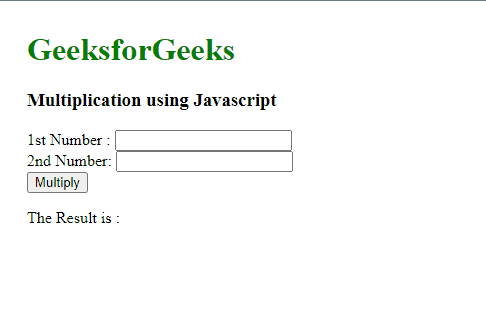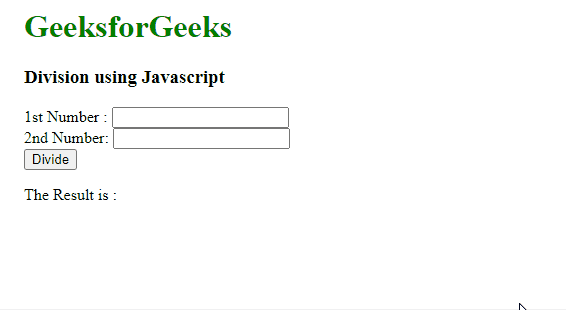Open In App

# How to calculate multiplication and division of two numbers using JavaScript ?

In this article, we will see the calculation such as multiplication and division using Javascript. An arithmetic operation operates on two numbers and numbers are called operands.

Multiplication The multiplication operator (*) multiplies two or more numbers and returns the product of the operands.

Syntax:

`a*b`

Example:

```let a =15;
let b = 12;
let c = a × b;```

Approach: Create the HTML form to take input from the user to perform multiplication operations. Add javascript code inside html to perform multiplication logic. Document.getElementById(id).value property returns the value of the value attribute of a text field.

Example: In this example, we will use the multiplication operator to multiply two numbers.

## HTML

 `<``body` `style``=``"margin: 30px"``>``    ``<``h1` `style``=``"color:green"``>GeeksforGeeks``    ``<``h3``>Multiplication using Javascript``    ``<``form``>``        ``1st Number : <``input` `type``=``"text"` `id``=``"firstNumber"` `/><``br``>``        ``2nd Number: <``input` `type``=``"text"` `id``=``"secondNumber"` `/><``br``>``        ``<``input` `type``=``"button"` `onClick``=``"multiplyBy()"` `Value``=``"Multiply"` `/><``br``>``    ``` `    ``<``p``>The Result is : <``br``>``        ``<``span` `id``=``"result"``>``    ```  `    ``<``script``>``        ``function multiplyBy()``        ``{``          ``num1 = document.getElementById(``            ``"firstNumber").value;``          ``num2 = document.getElementById(``            ``"secondNumber").value;``          ``document.getElementById(``            ``"result").innerHTML = num1 * num2;``        ``}``    `````

Output:Division The division operator (/) divides two or more numbers. the divided operand is known as the “dividend,” while the dividing operand is referred to as the “divisor.” The “quotient” is the value that remains after division.

Syntax:

`a / b`

Example:

```let a = 50;
let b = 20;
let c = a / b;```

Approach: Create the HTML form to take input from the user to perform division operations. Add JavaScript code inside HTML to perform division logic. Document.getElementById(id).value property returns the value of the value attribute of a text field.

Example: Below is the implementation of the above approach:

## HTML

 `<``body` `style``=``"margin: 30px"``>``    ``<``h1` `style``=``"color:green"``>GeeksforGeeks``    ``<``h3``>Division using Javascript``    ``<``form``>``        ``1st Number : <``input` `type``=``"text"` `id``=``"firstNumber"` `/><``br``>``        ``2nd Number: <``input` `type``=``"text"` `id``=``"secondNumber"` `/><``br``>``        ``<``input` `type``=``"button"` `onClick``=``"divideBy()"` `Value``=``"Divide"` `/>``    ``` `    ``<``p``>The Result is : <``br``>``        ``<``span` `id``=``"result"``>``    ```  `    ``<``script``>``        ``function divideBy()``        ``{``          ``num1 = document.getElementById(``            ``"firstNumber").value;``          ``num2 = document.getElementById(``            ``"secondNumber").value;``          ``document.getElementById(``            ``"result").innerHTML = num1 / num2;``        ``}``    `````

Output:multiplication and division of two numbers using JavaScript